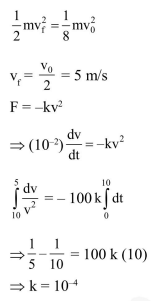Deepak Scored 45->99%ile with Bounce Back Crack Course. You can do it too!

# A body of mass

Question:

A body of mass $\mathrm{m}=10^{-2} \mathrm{~kg}$ is moving in a medium and experiences a frictional force $\mathrm{F}=-\mathrm{kv}^{2}$. Its intial speed is $\mathrm{v}_{0}=10 \mathrm{~ms}^{-1}$. If, after $10 \mathrm{~s}$, its energy is $\frac{1}{8} \mathrm{mv}_{0}^{2}$, the value of $\mathrm{k}$ will be :-

1. $10^{-4} \mathrm{~kg} \mathrm{~m} \mathrm{~m}^{-1}$

2. $10^{-1} \mathrm{~kg} \mathrm{~m}^{-1} \mathrm{~s}^{-1}$

3. $10^{-3} \mathrm{~kg} \mathrm{~m}^{-1}$

4. $10^{-3} \mathrm{~kg} \mathrm{~s}^{-1}$

Correct Option: 1

Solution: